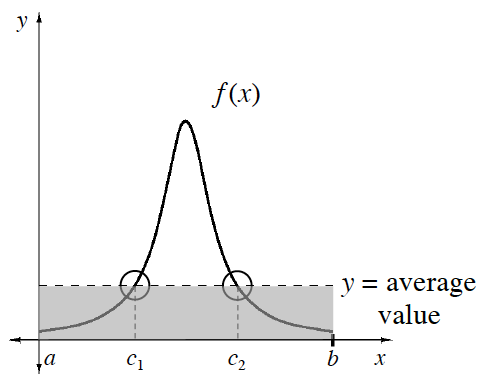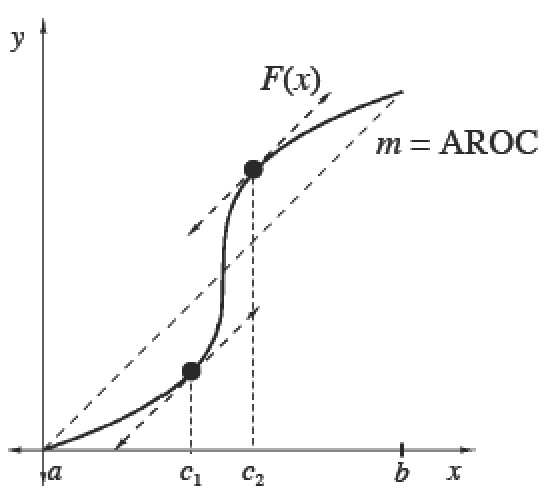### Home > CALC3RD > Chapter Ch7 > Lesson 7.1.5 > Problem7-51

7-51.

The point from problem 7-19 travels along the $x$-axis so that at time $t$ its position is given by $s(t) = t^3 - 5t^2 + 4t$, where $0 ≤ t ≤ 5$. Calculate the average velocity of the point. At what time(s) during the interval was the point traveling at this average velocity?

This is an application of the Mean Value Theorem of $f(x)$ (given $F(x)$).
In other words, where on the interval $[0,5]$is the slope of the tangent the same as the slope of the secant?

Determine where IROC $=$ AROC.

$v(t)=s'(t)=3t^{2}-10t+4=\frac{s(5)-s(0)}{5-0}$

real velocity $=$ average velocity
slope of tangent $=$ slope of secant
Solve for $t$ to find out WHERE the equation above holds true.

The Mean Value Theorem

 The Mean Value Theorem for IntegralsIf $f$ is continuous on $[a, b]$, then there exists at least one point $x = c$ in $\left(a, b\right)$ such that $\frac { 1 } { b - a } \int _ { a } ^ { b } f ( x ) d x= f(c)$.The Mean Value Theorem for DerivativesIf $F$ is continuous on $[a, b]$ and differentiable on $\left(a, b\right)$, then there exists at least one point $x = c$ in $\left(a, b\right)$ such that $F ^ { \prime } ( c ) = \frac { F ( b ) - F ( a ) } { b - a } = f ( c )$.